Precalculus 2e

# Chapter 7

### 7.1Solving Trigonometric Equations with Identities

1.
2.
3.

$sin 2 θ−1 tanθsinθ−tanθ = ( sinθ+1 )( sinθ−1 ) tanθ( sinθ−1 ) = sinθ+1 tanθ sin 2 θ−1 tanθsinθ−tanθ = ( sinθ+1 )( sinθ−1 ) tanθ( sinθ−1 ) = sinθ+1 tanθ$

4.

This is a difference of squares formula: $25−9 sin 2 θ=(5−3sinθ)(5+3sinθ). 25−9 sin 2 θ=(5−3sinθ)(5+3sinθ).$

5.

### 7.2Sum and Difference Identities

1.

$2 + 6 4 2 + 6 4$

2.

$2 − 6 4 2 − 6 4$

3.

$1− 3 1+ 3 1− 3 1+ 3$

4.

$cos( 5π 14 ) cos( 5π 14 )$

5.

### 7.3Double-Angle, Half-Angle, and Reduction Formulas

1.

$cos( 2α )= 7 32 cos( 2α )= 7 32$

2.

$cos 4 θ− sin 4 θ=( cos 2 θ+ sin 2 θ )( cos 2 θ− sin 2 θ )=cos( 2θ ) cos 4 θ− sin 4 θ=( cos 2 θ+ sin 2 θ )( cos 2 θ− sin 2 θ )=cos( 2θ )$

3.

$cos( 2θ )cosθ=( cos 2 θ− sin 2 θ )cosθ= cos 3 θ−cosθ sin 2 θ cos( 2θ )cosθ=( cos 2 θ− sin 2 θ )cosθ= cos 3 θ−cosθ sin 2 θ$

4.

5.

$− 2 5 − 2 5$

### 7.4Sum-to-Product and Product-to-Sum Formulas

1.

$1 2 ( cos6θ+cos2θ ) 1 2 ( cos6θ+cos2θ )$

2.

$1 2 ( sin2x+sin2y ) 1 2 ( sin2x+sin2y )$

3.

$−2− 3 4 −2− 3 4$

4.

$2sin( 2θ )cos( θ ) 2sin( 2θ )cos( θ )$

5.

$tanθcotθ− cos 2 θ=( sinθ cosθ )( cosθ sinθ )− cos 2 θ =1− cos 2 θ = sin 2 θ tanθcotθ− cos 2 θ=( sinθ cosθ )( cosθ sinθ )− cos 2 θ =1− cos 2 θ = sin 2 θ$

### 7.5Solving Trigonometric Equations

1.

$x= 7π 6 , 11π 6 x= 7π 6 , 11π 6$

2.

$π 3 ±πk π 3 ±πk$

3.

$θ≈1.7722±2πk θ≈1.7722±2πk$ and $θ≈4.5110±2πk θ≈4.5110±2πk$

4.

$cosθ=−1,θ=π cosθ=−1,θ=π$

5.

$π 2 , 2π 3 , 4π 3 , 3π 2 π 2 , 2π 3 , 4π 3 , 3π 2$

### 7.6Modeling with Trigonometric Functions

1.

The amplitude is $3, 3,$ and the period is $2 3 . 2 3 .$

2.
x $3sin( 3x ) 3sin( 3x )$
0 0
$π 6 π 6$ 3
$π 3 π 3$ 0
$π 2 π 2$ $−3 −3$
$2π 3 2π 3$ 03.

$y=8sin( π 12 t )+32 y=8sin( π 12 t )+32$
The temperature reaches freezing at noon and at midnight.4.

initial displacement =6, damping constant = -6, frequency = $2 π 2 π$

5.

$y=10 e −0.5t cos( πt ) y=10 e −0.5t cos( πt )$

6.

$y=5cos( 6πt ) y=5cos( 6πt )$

### 7.1 Section Exercises

1.

All three functions, $F F$, $GG$, and $H, H,$ are even.

This is because $F( −x )=sin( −x )sin( −x )=( −sinx )( −sinx )= sin 2 x=F( x ),G( −x )=cos( −x )cos( −x )=cosxcosx= cos 2 x=G( x ) F( −x )=sin( −x )sin( −x )=( −sinx )( −sinx )= sin 2 x=F( x ),G( −x )=cos( −x )cos( −x )=cosxcosx= cos 2 x=G( x )$ and $H( −x )=tan( −x )tan( −x )=( −tanx )( −tanx )= tan 2 x=H( x ). H( −x )=tan( −x )tan( −x )=( −tanx )( −tanx )= tan 2 x=H( x ).$

3.

When $cost=0, cost=0,$ then $sect= 1 0 , sect= 1 0 ,$ which is undefined.

5.

$sinx sinx$

7.

$secx secx$

9.

$csct csct$

11.

$−1 −1$

13.

$sec 2 x sec 2 x$

15.

$sin 2 x+1 sin 2 x+1$

17.

$1 sinx 1 sinx$

19.

$1 cotx 1 cotx$

21.

$tanx tanx$

23.

$−4secxtanx −4secxtanx$

25.

$± 1 cot 2 x +1 ± 1 cot 2 x +1$

27.

$± 1− sin 2 x sinx ± 1− sin 2 x sinx$

29.

$cosx− cos 3 x=cosx( 1− cos 2 x ) cosx− cos 3 x=cosx( 1− cos 2 x )$
$=cosx sin 2 x =cosx sin 2 x$

31.

$1+ sin 2 x cos 2 x = 1 cos 2 x + sin 2 x cos 2 x = sec 2 x+ tan 2 x= tan 2 x+1+ tan 2 x=1+2 tan 2 x 1+ sin 2 x cos 2 x = 1 cos 2 x + sin 2 x cos 2 x = sec 2 x+ tan 2 x= tan 2 x+1+ tan 2 x=1+2 tan 2 x$

33.

$cos 2 x− tan 2 x=1− sin 2 x−( sec 2 x−1 )=1− sin 2 x− sec 2 x+1=2− sin 2 x− sec 2 x cos 2 x− tan 2 x=1− sin 2 x−( sec 2 x−1 )=1− sin 2 x− sec 2 x+1=2− sin 2 x− sec 2 x$

35.

False

37.

False

39.

Proved with negative and Pythagorean Identities

41.

True $3 sin 2 θ+4 cos 2 θ=3 sin 2 θ+3 cos 2 θ+ cos 2 θ=3( sin 2 θ+ cos 2 θ )+ cos 2 θ=3+ cos 2 θ 3 sin 2 θ+4 cos 2 θ=3 sin 2 θ+3 cos 2 θ+ cos 2 θ=3( sin 2 θ+ cos 2 θ )+ cos 2 θ=3+ cos 2 θ$

### 7.2 Section Exercises

1.

The cofunction identities apply to complementary angles. Viewing the two acute angles of a right triangle, if one of those angles measures $x, x,$ the second angle measures $π 2 −x. π 2 −x.$ Then $sinx=cos( π 2 −x ). sinx=cos( π 2 −x ).$ The same holds for the other cofunction identities. The key is that the angles are complementary.

3.

$sin( −x )=−sinx, sin( −x )=−sinx,$ so $sinx sinx$ is odd. $cos( −x )=cos( 0−x )=cosx, cos( −x )=cos( 0−x )=cosx,$ so $cosx cosx$ is even.

5.

$2 + 6 4 2 + 6 4$

7.

$6 − 2 4 6 − 2 4$

9.

$−2− 3 −2− 3$

11.

$− 2 2 sinx− 2 2 cosx − 2 2 sinx− 2 2 cosx$

13.

$− 1 2 cosx− 3 2 sinx − 1 2 cosx− 3 2 sinx$

15.

$cscθ cscθ$

17.

$cotx cotx$

19.

$tan( x 10 ) tan( x 10 )$

21.

$sin(a−b)=( 4 5 )( 1 3 )−( 3 5 )( 2 2 3 )= 4−6 2 15 sin(a−b)=( 4 5 )( 1 3 )−( 3 5 )( 2 2 3 )= 4−6 2 15$
$cos(a+b)=( 3 5 )( 1 3 )−( 4 5 )( 2 2 3 )= 3−8 2 15 cos(a+b)=( 3 5 )( 1 3 )−( 4 5 )( 2 2 3 )= 3−8 2 15$

23.

$2 − 6 4 2 − 6 4$

25.

$sinx sinx$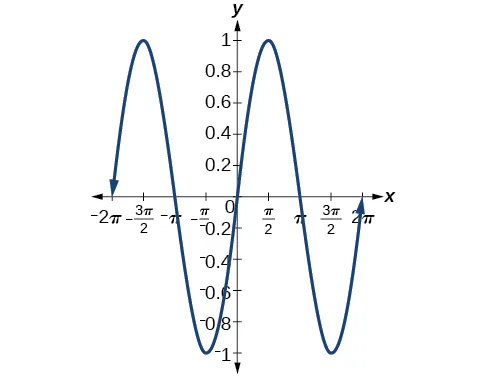27.

$cot( π 6 −x ) cot( π 6 −x )$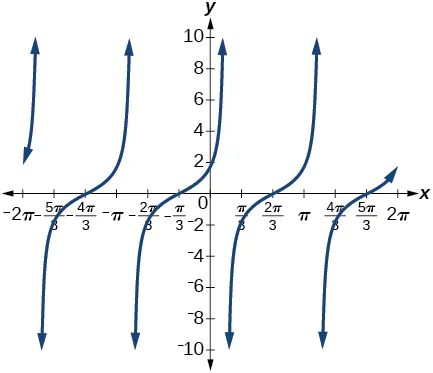29.

$cot( π 4 +x ) cot( π 4 +x )$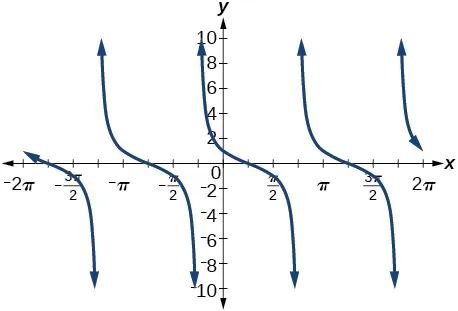31.

$sinx 2 + cosx 2 sinx 2 + cosx 2$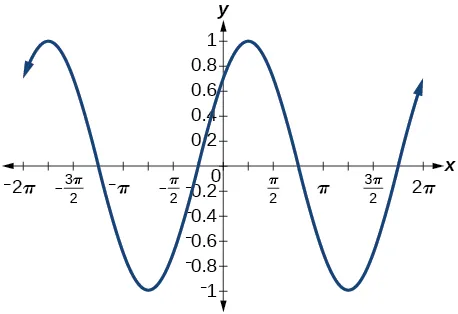33.

They are the same.

35.

They are the different, try $g( x )=sin( 9x )−cos( 3x )sin( 6x ). g( x )=sin( 9x )−cos( 3x )sin( 6x ).$

37.

They are the same.

39.

They are the different, try $g( θ )= 2tanθ 1− tan 2 θ . g( θ )= 2tanθ 1− tan 2 θ .$

41.

They are different, try $g( x )= tanx−tan( 2x ) 1+tanxtan( 2x ) . g( x )= tanx−tan( 2x ) 1+tanxtan( 2x ) .$

43.

45.

$1+ 3 2 2 , 1+ 3 2 2 ,$ or 0.9659

47.

$tan( x+ π 4 )= tanx+tan( π 4 ) 1−tanxtan( π 4 ) = tanx+1 1−tanx(1) = tanx+1 1−tanx tan( x+ π 4 )= tanx+tan( π 4 ) 1−tanxtan( π 4 ) = tanx+1 1−tanx(1) = tanx+1 1−tanx$

49.

$cos( a+b ) cosacosb = cosacosb cosacosb − sinasinb cosacosb =1−tanatanb cos( a+b ) cosacosb = cosacosb cosacosb − sinasinb cosacosb =1−tanatanb$

51.

$cos( x+h )−cosx h = cosxcosh−sinxsinh−cosx h = cosx(cosh−1)−sinxsinh h =cosx cosh−1 h −sinx sinh h cos( x+h )−cosx h = cosxcosh−sinxsinh−cosx h = cosx(cosh−1)−sinxsinh h =cosx cosh−1 h −sinx sinh h$

53.

True

55.

True. Note that $sin( α+β )=sin( π−γ ) sin( α+β )=sin( π−γ )$ and expand the right hand side.

### 7.3 Section Exercises

1.

Use the Pythagorean identities and isolate the squared term.

3.

$1−cosx sinx , sinx 1+cosx , 1−cosx sinx , sinx 1+cosx ,$ multiplying the top and bottom by $1−cosx 1−cosx$ and $1+cosx , 1+cosx ,$ respectively.

5.

a) $3 7 32 3 7 32$ b) $31 32 31 32$ c) $3 7 31 3 7 31$

7.

a) $3 2 3 2$ b) $− 1 2 − 1 2$ c) $− 3 − 3$

9.

$cosθ=− 2 5 5 ,sinθ= 5 5 ,tanθ=− 1 2 ,cscθ= 5 ,secθ=− 5 2 ,cotθ=−2 cosθ=− 2 5 5 ,sinθ= 5 5 ,tanθ=− 1 2 ,cscθ= 5 ,secθ=− 5 2 ,cotθ=−2$

11.

$2sin( π 2 ) 2sin( π 2 )$

13.

$2− 2 2 2− 2 2$

15.

$2− 3 2 2− 3 2$

17.

$2+ 3 2+ 3$

19.

$−1− 2 −1− 2$

21.

a) $3 13 13 3 13 13$ b) $− 2 13 13 − 2 13 13$ c) $− 3 2 − 3 2$

23.

a) $10 4 10 4$ b) $6 4 6 4$ c) $15 3 15 3$

25.

$120 169 ,– 119 169 ,– 120 119 120 169 ,– 119 169 ,– 120 119$

27.

$2 13 13 , 3 13 13 , 2 3 2 13 13 , 3 13 13 , 2 3$

29.

$cos( 74 ∘ ) cos( 74 ∘ )$

31.

$cos(18x) cos(18x)$

33.

$3sin(10x) 3sin(10x)$

35.

$−2sin( −x )cos( −x )=−2(−sin( x )cos( x ))=sin( 2x ) −2sin( −x )cos( −x )=−2(−sin( x )cos( x ))=sin( 2x )$

37.

$sin( 2θ ) 1+cos( 2θ ) tan 2 θ= 2sin( θ )cos( θ ) 1+ cos 2 θ− sin 2 θ tan 2 θ= 2sin( θ )cos( θ ) 2 cos 2 θ tan 2 θ= sin( θ ) cosθ tan 2 θ= tan θ tan 2 θ=tan3θ sin( 2θ ) 1+cos( 2θ ) tan 2 θ= 2sin( θ )cos( θ ) 1+ cos 2 θ− sin 2 θ tan 2 θ= 2sin( θ )cos( θ ) 2 cos 2 θ tan 2 θ= sin( θ ) cosθ tan 2 θ= tan θ tan 2 θ=tan3θ$

39.

$1+cos(12x) 2 1+cos(12x) 2$

41.

$3+cos(12x)−4cos(6x) 8 3+cos(12x)−4cos(6x) 8$

43.

$2+cos(2x)−2cos(4x)−cos(6x) 32 2+cos(2x)−2cos(4x)−cos(6x) 32$

45.

$3+cos(4x)−4cos(2x) 3+cos(4x)+4cos(2x) 3+cos(4x)−4cos(2x) 3+cos(4x)+4cos(2x)$

47.

$1−cos(4x) 8 1−cos(4x) 8$

49.

$3+cos(4x)−4cos(2x) 4(cos(2x)+1) 3+cos(4x)−4cos(2x) 4(cos(2x)+1)$

51.

$( 1+cos( 4x ) )sinx 2 ( 1+cos( 4x ) )sinx 2$

53.

$4sinxcosx( cos 2 x− sin 2 x ) 4sinxcosx( cos 2 x− sin 2 x )$

55.

$2tanx 1+ tan 2 x = 2sinx cosx 1+ sin 2 x cos 2 x = 2sinx cosx cos 2 x+ sin 2 x cos 2 x = 2tanx 1+ tan 2 x = 2sinx cosx 1+ sin 2 x cos 2 x = 2sinx cosx cos 2 x+ sin 2 x cos 2 x =$
$2sinx cosx . cos 2 x 1 =2sinxcosx=sin(2x) 2sinx cosx . cos 2 x 1 =2sinxcosx=sin(2x)$

57.

$2sinxcosx 2 cos 2 x−1 = sin(2x) cos(2x) =tan(2x) 2sinxcosx 2 cos 2 x−1 = sin(2x) cos(2x) =tan(2x)$

59.

$sin(x+2x)=sinxcos(2x)+sin(2x)cosx =sinx( cos 2 x− sin 2 x)+2sinxcosxcosx =sinx cos 2 x− sin 3 x+2sinx cos 2 x =3sinx cos 2 x− sin 3 x sin(x+2x)=sinxcos(2x)+sin(2x)cosx =sinx( cos 2 x− sin 2 x)+2sinxcosxcosx =sinx cos 2 x− sin 3 x+2sinx cos 2 x =3sinx cos 2 x− sin 3 x$

61.

$1+cos(2t) sin(2t)−cost = 1+2 cos 2 t−1 2sintcost−cost = 2 cos 2 t cost(2sint−1) = 2cost 2sint−1 1+cos(2t) sin(2t)−cost = 1+2 cos 2 t−1 2sintcost−cost = 2 cos 2 t cost(2sint−1) = 2cost 2sint−1$

63.

$( cos 2 (4x)− sin 2 (4x)−sin(8x))( cos 2 (4x)− sin 2 (4x)+sin(8x) )= =( cos(8x)−sin(8x))(cos(8x)+sin(8x) ) = cos 2 (8x)− sin 2 (8x) =cos(16x) ( cos 2 (4x)− sin 2 (4x)−sin(8x))( cos 2 (4x)− sin 2 (4x)+sin(8x) )= =( cos(8x)−sin(8x))(cos(8x)+sin(8x) ) = cos 2 (8x)− sin 2 (8x) =cos(16x)$

### 7.4 Section Exercises

1.

Substitute $α α$ into cosine and $β β$ into sine and evaluate.

3.

Answers will vary. There are some equations that involve a sum of two trig expressions where when converted to a product are easier to solve. For example: $sin(3x)+sinx cosx =1. sin(3x)+sinx cosx =1.$ When converting the numerator to a product the equation becomes: $2sin(2x)cosx cosx =1 2sin(2x)cosx cosx =1$

5.

$8( cos( 5x )−cos( 27x ) ) 8( cos( 5x )−cos( 27x ) )$

7.

$sin( 2x )+sin( 8x ) sin( 2x )+sin( 8x )$

9.

$1 2 ( cos( 6x )−cos( 4x ) ) 1 2 ( cos( 6x )−cos( 4x ) )$

11.

$2cos( 5t )cost 2cos( 5t )cost$

13.

$2cos( 7x ) 2cos( 7x )$

15.

$2cos( 6x )cos( 3x ) 2cos( 6x )cos( 3x )$

17.

$1 4 ( 1+ 3 ) 1 4 ( 1+ 3 )$

19.

$1 4 ( 3 −2 ) 1 4 ( 3 −2 )$

21.

$1 4 ( 3 −1 ) 1 4 ( 3 −1 )$

23.

$cos( 80° )−cos( 120° ) cos( 80° )−cos( 120° )$

25.

$1 2 (sin(221°)+sin(205°)) 1 2 (sin(221°)+sin(205°))$

27.

$2 cos( 31° ) 2 cos( 31° )$

29.

$2cos(66.5°)sin(34.5°) 2cos(66.5°)sin(34.5°)$

31.

$2sin( −1.5° )cos( 0.5° ) 2sin( −1.5° )cos( 0.5° )$

33.

$2sin(7x)−2sinx=2sin(4x+3x)−2sin(4x−3x)= 2(sin(4x)cos(3x)+sin(3x)cos(4x))−2(sin(4x)cos(3x)−sin(3x)cos(4x))= 2sin(4x)cos(3x)+2sin(3x)cos(4x))−2sin(4x)cos(3x)+2sin(3x)cos(4x))= 4sin(3x)cos(4x) 2sin(7x)−2sinx=2sin(4x+3x)−2sin(4x−3x)= 2(sin(4x)cos(3x)+sin(3x)cos(4x))−2(sin(4x)cos(3x)−sin(3x)cos(4x))= 2sin(4x)cos(3x)+2sin(3x)cos(4x))−2sin(4x)cos(3x)+2sin(3x)cos(4x))= 4sin(3x)cos(4x)$

35.

$sinx+sin( 3x )=2sin( 4x 2 )cos( −2x 2 )= sinx+sin( 3x )=2sin( 4x 2 )cos( −2x 2 )=$
$2sin(2x)cosx=2(2sinxcosx)cosx= 2sin(2x)cosx=2(2sinxcosx)cosx=$
$4sinx cos 2 x 4sinx cos 2 x$

37.

$2tanxcos( 3x )= 2sinxcos(3x) cosx = 2(.5(sin(4x)−sin(2x))) cosx 2tanxcos( 3x )= 2sinxcos(3x) cosx = 2(.5(sin(4x)−sin(2x))) cosx$
$= 1 cosx ( sin(4x)−sin(2x) )=secx( sin( 4x )−sin( 2x ) ) = 1 cosx ( sin(4x)−sin(2x) )=secx( sin( 4x )−sin( 2x ) )$

39.

41.

$−2sin( 33 ∘ )sin( 11 ∘ ),−0.2078 −2sin( 33 ∘ )sin( 11 ∘ ),−0.2078$

43.

$1 2 ( cos( 99 ∘ )−cos( 71 ∘ ) ),−0.2410 1 2 ( cos( 99 ∘ )−cos( 71 ∘ ) ),−0.2410$

45.

It is and identity.

47.

It is not an identity, but $2 cos 3 x 2 cos 3 x$ is.

49.

$tan( 3t ) tan( 3t )$

51.

$2cos( 2x ) 2cos( 2x )$

53.

$−sin(14x) −sin(14x)$

55.

Start with $cosx+cosy. cosx+cosy.$ Make a substitution and let $x=α+β x=α+β$ and let $y=α−β, y=α−β,$ so $cosx+cosy cosx+cosy$ becomes
$cos(α+β)+cos(α−β)=cosαcosβ−sinαsinβ+cosαcosβ+sinαsinβ=2cosαcosβcos(α+β)+cos(α−β)=cosαcosβ−sinαsinβ+cosαcosβ+sinαsinβ=2cosαcosβ$

Since $x=α+β x=α+β$ and $y=α−β, y=α−β,$ we can solve for $α α$ and $β β$ in terms of x and y and substitute in for $2cosαcosβ 2cosαcosβ$ and get $2cos( x+y 2 )cos( x−y 2 ). 2cos( x+y 2 )cos( x−y 2 ).$

57.

$cos( 3x )+cosx cos( 3x )−cosx = 2cos( 2x )cosx −2sin( 2x )sinx =−cot( 2x )cotx cos( 3x )+cosx cos( 3x )−cosx = 2cos( 2x )cosx −2sin( 2x )sinx =−cot( 2x )cotx$

59.

$cos( 2y )−cos( 4y ) sin( 2y )+sin( 4y ) = −2sin( 3y )sin( −y ) 2sin( 3y )cosy = 2sin( 3y )sin( y ) 2sin( 3y )cosy =tany cos( 2y )−cos( 4y ) sin( 2y )+sin( 4y ) = −2sin( 3y )sin( −y ) 2sin( 3y )cosy = 2sin( 3y )sin( y ) 2sin( 3y )cosy =tany$

61.

$cosx−cos( 3x )=−2sin(2x)sin(−x)= 2(2sinxcosx)sinx=4 sin 2 xcosx cosx−cos( 3x )=−2sin(2x)sin(−x)= 2(2sinxcosx)sinx=4 sin 2 xcosx$

63.

$tan( π 4 −t )= tan( π 4 )−tant 1+tan( π 4 )tan(t) = 1−tant 1+tant tan( π 4 −t )= tan( π 4 )−tant 1+tan( π 4 )tan(t) = 1−tant 1+tant$

### 7.5 Section Exercises

1.

There will not always be solutions to trigonometric function equations. For a basic example, $cos(x)=−5. cos(x)=−5.$

3.

If the sine or cosine function has a coefficient of one, isolate the term on one side of the equals sign. If the number it is set equal to has an absolute value less than or equal to one, the equation has solutions, otherwise it does not. If the sine or cosine does not have a coefficient equal to one, still isolate the term but then divide both sides of the equation by the leading coefficient. Then, if the number it is set equal to has an absolute value greater than one, the equation has no solution.

5.

$π 3 , 2π 3 π 3 , 2π 3$

7.

$3π 4 , 5π 4 3π 4 , 5π 4$

9.

$π 4 , 5π 4 π 4 , 5π 4$

11.

$π 4 , 3π 4 , 5π 4 , 7π 4 π 4 , 3π 4 , 5π 4 , 7π 4$

13.

$π 4 , 7π 4 π 4 , 7π 4$

15.

$7π 6 , 11π 6 7π 6 , 11π 6$

17.

$π 18 π 18$, $5π 18 5π 18$, $13π 18 13π 18$, $17π 18 17π 18$, $25π 18 25π 18$, $29π 18 29π 18$

19.

$3π 12 , 5π 12 , 11π 12 , 13π 12 , 19π 12 , 21π 12 3π 12 , 5π 12 , 11π 12 , 13π 12 , 19π 12 , 21π 12$

21.

$1 6 , 5 6 , 13 6 , 17 6 , 25 6 , 29 6 , 37 6 1 6 , 5 6 , 13 6 , 17 6 , 25 6 , 29 6 , 37 6$

23.

$0, π 3 ,π, 5π 3 0, π 3 ,π, 5π 3$

25.

$π 3 ,π, 5π 3 π 3 ,π, 5π 3$

27.

$π 3 , 3π 2 , 5π 3 π 3 , 3π 2 , 5π 3$

29.

$0,π 0,π$

31.

$π− sin −1 ( − 1 4 ), 7π 6 , 11π 6 ,2π+ sin −1 ( − 1 4 ) π− sin −1 ( − 1 4 ), 7π 6 , 11π 6 ,2π+ sin −1 ( − 1 4 )$

33.

$1 3 ( sin −1 ( 9 10 ) ), 1 3 ( sin −1 ( 9 10 ) ),$ $π 3 − 1 3 ( sin −1 ( 9 10 ) ), π 3 − 1 3 ( sin −1 ( 9 10 ) ),$ $2π 3 + 1 3 ( sin −1 ( 9 10 ) ), 2π 3 + 1 3 ( sin −1 ( 9 10 ) ),$ $π− 1 3 ( sin −1 ( 9 10 ) ), π− 1 3 ( sin −1 ( 9 10 ) ),$ $4π 3 + 1 3 ( sin −1 ( 9 10 ) ), 4π 3 + 1 3 ( sin −1 ( 9 10 ) ),$ $5π 3 − 1 3 ( sin −1 ( 9 10 ) ) 5π 3 − 1 3 ( sin −1 ( 9 10 ) )$

35.

$0 0$

37.

$θ=sin-123,θ=sin-123,$ $π-sin-123,π-sin-123,$ $π+sin-123,π+sin-123,$ $2π-sin-1232π-sin-123$

39.

$3π 2 , π 6 , 5π 6 3π 2 , π 6 , 5π 6$

41.

$0, π 3 ,π, 4π 3 0, π 3 ,π, 4π 3$

43.

There are no solutions.

45.

$cos −1 ( 1 3 ( 1− 7 ) ),2π− cos −1 ( 1 3 ( 1− 7 ) ) cos −1 ( 1 3 ( 1− 7 ) ),2π− cos −1 ( 1 3 ( 1− 7 ) )$

47.

$tan −1 ( 1 2 ( 29 −5 ) ),π+ tan −1 ( 1 2 ( − 29 −5 ) ),π+ tan −1 ( 1 2 ( 29 −5 ) ),2π+ tan −1 ( 1 2 ( − 29 −5 ) ) tan −1 ( 1 2 ( 29 −5 ) ),π+ tan −1 ( 1 2 ( − 29 −5 ) ),π+ tan −1 ( 1 2 ( 29 −5 ) ),2π+ tan −1 ( 1 2 ( − 29 −5 ) )$

49.

There are no solutions.

51.

There are no solutions.

53.

$0, 2π 3 , 4π 3 0, 2π 3 , 4π 3$

55.

$π 4 , 3π 4 , 5π 4 , 7π 4 π 4 , 3π 4 , 5π 4 , 7π 4$

57.

$sin −1 ( 3 5 ), π 2 ,π− sin −1 ( 3 5 ), 3π 2 sin −1 ( 3 5 ), π 2 ,π− sin −1 ( 3 5 ), 3π 2$

59.

$cos −1 ( − 1 4 ) cos −1 ( − 1 4 )$, $2π− cos −1 ( − 1 4 ) 2π− cos −1 ( − 1 4 )$

61.

$π 3 , cos −1 ( − 3 4 ),2π− cos −1 ( − 3 4 ), 5π 3 π 3 , cos −1 ( − 3 4 ),2π− cos −1 ( − 3 4 ), 5π 3$

63.

$cos −1 ( 3 4 ), cos −1 ( − 2 3 ),2π− cos −1 ( − 2 3 ) cos −1 ( 3 4 ), cos −1 ( − 2 3 ),2π− cos −1 ( − 2 3 )$, $2π− cos −1 ( 3 4 ) 2π− cos −1 ( 3 4 )$

65.

$0, π 2 ,π, 3π 2 0, π 2 ,π, 3π 2$

67.

$π 3 , cos −1 ( − 1 4 ),2π− cos −1 ( − 1 4 ), 5π 3 π 3 , cos −1 ( − 1 4 ),2π− cos −1 ( − 1 4 ), 5π 3$

69.

There are no solutions.

71.

$π+ tan −1 ( −2 ) π+ tan −1 ( −2 )$, $π+ tan −1 ( − 3 2 ),π+ tan −1 ( − 3 2 ),$ $2π+ tan −1 ( −2 ),2π+ tan −1 ( −2 ),$ $2π+ tan −1 ( − 3 2 ) 2π+ tan −1 ( − 3 2 )$

73.

$2πk+0.2734,2πk+2.8682 2πk+0.2734,2πk+2.8682$

75.

$πk−0.3277 πk−0.3277$

77.

$0.6694,1.8287,3.8110,4.9703 0.6694,1.8287,3.8110,4.9703$

79.

$1.0472,3.1416,5.2360 1.0472,3.1416,5.2360$

81.

$0.5326,1.7648,3.6742,4.9064 0.5326,1.7648,3.6742,4.9064$

83.

$sin −1 ( 1 4 ),π− sin −1 ( 1 4 ), 3π 2 sin −1 ( 1 4 ),π− sin −1 ( 1 4 ), 3π 2$

85.

$π 2 , 3π 2 π 2 , 3π 2$

87.

There are no solutions.

89.

$0, π 2 ,π, 3π 2 0, π 2 ,π, 3π 2$

91.

There are no solutions.

93.

$7.2 ∘ 7.2 ∘$

95.

$5.7 ∘ 5.7 ∘$

97.

$82.4 ∘ 82.4 ∘$

99.

$31.0 ∘ 31.0 ∘$

101.

$88.7 ∘ 88.7 ∘$

103.

$59.0 ∘ 59.0 ∘$

105.

$36.9 ∘ 36.9 ∘$

### 7.6 Section Exercises

1.

Physical behavior should be periodic, or cyclical.

3.

Since cumulative rainfall is always increasing, a sinusoidal function would not be ideal here.

5.

$y=−3cos( π 6 x )−1 y=−3cos( π 6 x )−1$

7.

$5sin(2x)+2 5sin(2x)+2$

8.

$y= 4- 6cos( xπ 2 ) y=4- 6cos( xπ 2 )$

10.

$y= tan( xπ 8 ) y= tan( xπ 8 )$

12.

$tan( xπ 12 ) tan( xπ 12 )$

13.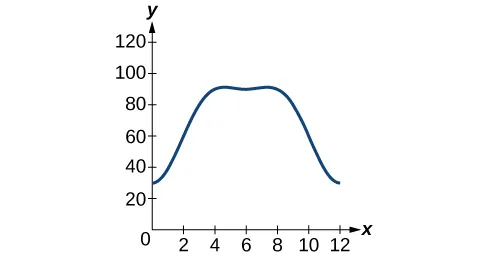15.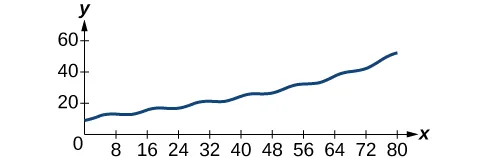17.

75 °F

19.

8 a.m.

21.

2:49

23.

From June 15 through November 16

25.

From day 41 through day 68

27.

Floods: April 16 to July 15. Drought: October 16 to January 15.

29.

Amplitude: 8, period: $1 3 , 1 3 ,$ frequency: 3 Hz

31.

Amplitude: 4, period: $4 , 4 ,$ frequency: $1 4 1 4$ Hz

33.

$P(t)=−19cos( π 6 t )+800+ 160 12 t P(t)=−19cos( π 6 t )+800+ 40 3 t P(t)=−19cos( π 6 t )+800+ 160 12 t P(t)=−19cos( π 6 t )+800+ 40 3 t$

35.

$P(t)=−33cos( π 6 t )+900+ ( 1.07 ) t P(t)=−33cos( π 6 t )+900+ ( 1.07 ) t$

37.

$D(t)=10 ( 0.85 ) t cos( 36πt ) D(t)=10 ( 0.85 ) t cos( 36πt )$

39.

$D(t)=17 ( 0.9145 ) t cos( 28πt ) D(t)=17 ( 0.9145 ) t cos( 28πt )$

41.

6 years

43.

15.4 seconds

45.

Spring 2 comes to rest first after 7.3 seconds.

47.

234.3 miles, at 72.2°

49.

$y=6 ( 4 ) x +5sin( π 2 x ) y=6 ( 4 ) x +5sin( π 2 x )$

51.

$y=4 (– 2 ) x +8sin( π 2 x ) y=4 (– 2 ) x +8sin( π 2 x )$

53.

$y=3 ( 2 ) x cos( π 2 x ) +1 y=3 ( 2 ) x cos( π 2 x ) +1$

### Review Exercises

1.

$sin −1 ( 3 3 ),π− sin −1 ( 3 3 ),π+ sin −1 ( 3 3 ),2π− sin −1 ( 3 3 ) sin −1 ( 3 3 ),π− sin −1 ( 3 3 ),π+ sin −1 ( 3 3 ),2π− sin −1 ( 3 3 )$

3.

$7π 6 , 11π 6 7π 6 , 11π 6$

5.

$sin −1 ( 1 4 ),π− sin −1 ( 1 4 ) sin −1 ( 1 4 ),π− sin −1 ( 1 4 )$

7.

$1 1$

9.

Yes

11.

$−2− 3 −2− 3$

13.

$2 2 2 2$

15.

17.

$tan( 5 8 x ) tan( 5 8 x )$

19.

$3 3 3 3$

21.

$− 24 25 ,− 7 25 , 24 7 − 24 25 ,− 7 25 , 24 7$

23.

$2( 2+ 2 ) 2( 2+ 2 )$

25.

$2 10 , 7 2 10 , 1 7 , 3 5 , 4 5 , 3 4 2 10 , 7 2 10 , 1 7 , 3 5 , 4 5 , 3 4$

27.

29.

$10sinx−5sin( 3x )+sin( 5x ) 8( cos( 2x )+1 ) 10sinx−5sin( 3x )+sin( 5x ) 8( cos( 2x )+1 )$

31.

$3 2 3 2$

33.

$− 2 2 − 2 2$

35.

$1 2 ( sin(6x)+sin(12x) ) 1 2 ( sin(6x)+sin(12x) )$

37.

$2sin( 13 2 x )cos( 9 2 x ) 2sin( 13 2 x )cos( 9 2 x )$

39.

$3π 4 , 7π 4 3π 4 , 7π 4$

41.

$0, π 6 , 5π 6 ,π 0, π 6 , 5π 6 ,π$

43.

$3π 2 3π 2$

45.

No solution

47.

$0.2527,2.8889,4.7124 0.2527,2.8889,4.7124$

49.

$1.3694 1.3694$, $1.91061.9106$, $4.37264.3726$, $4.9137 4.9137$

51.

$3sin( xπ 2 )−2 3sin( xπ 2 )−2$

53.

$71.6 ∘ 71.6 ∘$

55.

$P(t)=950−450sin( π 6 t ) P(t)=950−450sin( π 6 t )$

57.

Amplitude: 3, period: 2, frequency: $1 2 1 2$ Hz

59.

$C(t)=20sin(2πt)+100 (1.4427) t C(t)=20sin(2πt)+100 (1.4427) t$

### Practice Test

1.

1

3.

$2 − 6 4 2 − 6 4$

5.

$− 2 − 3 − 2 − 3$

7.

$0,π 0,π$

9.

$π 2 , 3π 2 π 2 , 3π 2$

11.

$2cos(3x)cos(5x)2cos(3x)cos(5x)$

13.

$x=cos–1 ( 1 5 ) x=cos–1 ( 1 5 )$

15.

$3 5 ,− 4 5 , − 3 4 3 5 ,− 4 5 , − 3 4$

17.

$tan3x–tan x sec2x =tanx(tan2x–sec2x) =tanx(tan2x–(1+tan2x)) =tanx(tan2x–1–tan2x) =–tanx=tan(–x)=tan–x) tan3x–tan x sec2x =tanx(tan2x–sec2x) =tanx(tan2x–(1+tan2x)) =tanx(tan2x–1–tan2x) =–tanx=tan(–x)=tan–x)$

19.

$sin(2x)sinx–cos(2x)cosx =2sin x cosxsinx–2cos2x–1cosx =2cosx–2cosx+1cosx = 1cosx=secx=secx sin(2x)sinx–cos(2x)cosx =2sin x cosxsinx–2cos2x–1cosx =2cosx–2cosx+1cosx = 1cosx=secx=secx$

21.

Amplitude: $14 14$ , period $160 160$ , frequency: 60 Hz

23.

Amplitude: $8 8$ , fast period: $1500 1500$ , fast frequency: 500 Hz, slow period: $110 110$ , slow frequency: 10 Hz

25.

$D(t)=20(0.9086)tcos(4πt) D(t)=20(0.9086)tcos(4πt)$, 31 seconds

Order a print copy

As an Amazon Associate we earn from qualifying purchases.